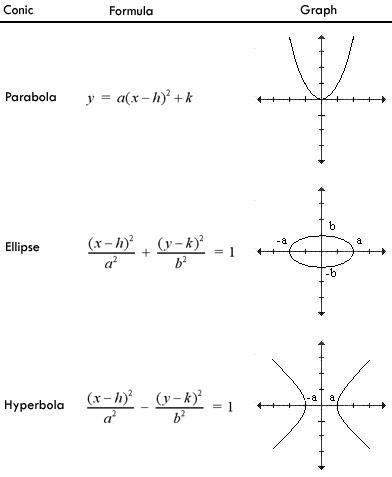# What is the difference between identifying a parabola, ellipse, hyperbola, and a circle?

Circle: ${\left(x - h\right)}^{2} + {\left(y - k\right)}^{2} = {r}^{2}$
parabola: ${\left(x - h\right)}^{2} = \left(y - k\right)$
ellipse: ${\left(x - h\right)}^{2} / {a}^{2} + {\left(y - k\right)}^{2} / b$
hyperbola: ${\left(x - h\right)}^{2} / {a}^{2} - {\left(y - k\right)}^{2} / {b}^{2} = 1$
() ()